All submissions of the EM system will be redirected to Online Manuscript Submission System. Authors are requested to submit articles directly to Online Manuscript Submission System of respective journal.

# Some Improved Ratio Estimators for Estimating Mean of Finite Population

1Department of Statistics, University Of Peshawar, Pakistan

2Department of Statistics, Islamia College Peshawar, Pakistan

Corresponding Author:
Department of Statistics, University of Peshawar, Pakistan
E-mail: [email protected]

Received date: 27/03/2018 Accepted date: 21/06/2018 Published date: 25/06/2018.

Visit for more related articles at Research & Reviews: Journal of Statistics and Mathematical Sciences

## Abstract

In this paper we have proposed an efficient estimator for estimating the mean of finite population under simple random sampling schemes. We have proposed a modified ratio estimator whose efficiency is the same as of regression estimator. It is a well establish fact that linear regression estimator is more efficient than most of the ratio estimators. We have found the Bias and MSE up to first order of approximation. The conditions under which the proposed estimators perform well as compared to other estimators. These properties are supported by real data sets.

#### Keywords

Auxiliary information, Study variable, Bias, MSE, Percentage relative efficiency

#### Introduction

The efficiency of an estimator can be increased largely, if we incorporate the auxiliary/benchmark variable(s) correlated with the study variable. Utilizing auxiliary information in a way so that the results become highly efficient. The use of auxiliary information is a challenging problem. Many statisticians use auxiliary information in their own way. It was Cochran who first uses auxiliary information in estimating the mean of finite population. Many other Statisticians make use of auxiliary information at estimation stages [1-6].

The Classical estimator of the mean of the finite populationThis estimator is an unbiasedestimator of population mean and its variance is given by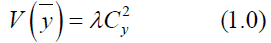Cochran  introduces the traditional ratio type estimator and is given by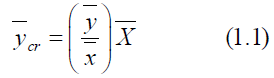The Bias and MSE are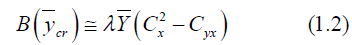And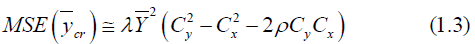Where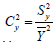the coefficient of variation of the study variable Y is,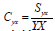is the coefficient ofvariation of the auxiliary variableis the coefficient of covariance between the studyvariable and the auxiliary variable and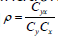is the coefficient of correlation between Y and X.Sisodia and Dwivedi introduces the ratio type estimator for estimating the mean of finitepopulation and is follows as,The mean square error is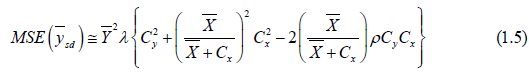An exponential ratio type estimator due to Bhal and Tuteja is given by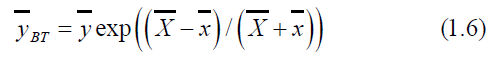The Bias and MSE of (1.6) is given by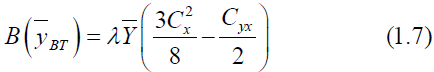&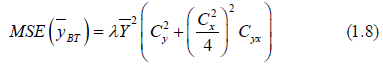Sing and tailor  proposed another estimator for estimating the mean of finite population for theknown value of correlation coefficient between the study variable and auxiliary variable. The estimatoris given by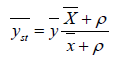(1.9)

The MSE is written as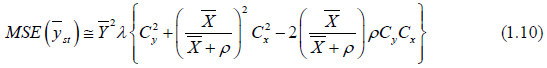#### Proposed Estimators

We suggest the following estimators(2.0)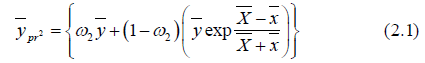Where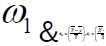are constants or some functions of auxiliary information which is to be determined, sothat to get minimum MSE for the proposed estimator.

Properties of the First Proposed Estimator

We will come across through the following terms and notations to compute the Bias and MSE for theproposed estimator,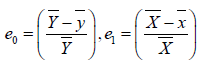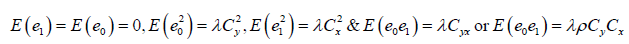Then we can write (2.0) as followsBy neglecting the higher power terms, we haveThe Bias corresponding to (2.0) is given by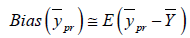Or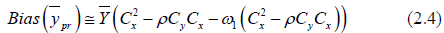For MSE, Squaring and taking expectation of equation (2.3), we have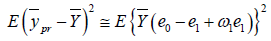(2.5)

Since,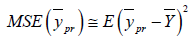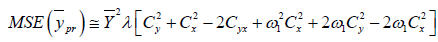(2.6)

We can find the optimum value ofby minimizing the MSE ofwith respect to ω1

Differentiating (2.6) w.r.to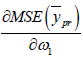and equating to zerowe get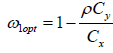By substituting, in (2.4) and (2.6) we getProperties of the Second Proposed Estimator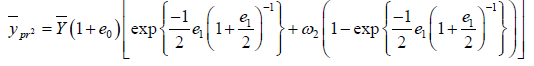(2.9)

Terms with power higher than two is ignored, we have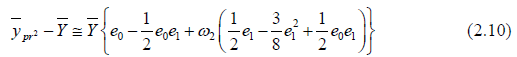We can write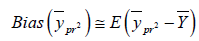orFor MSE, Squaring and taking expectation of equation (3.3), we have(2.12)

Since,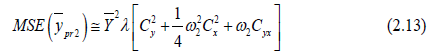The optimum value of ω2 can be find out by minimizing (3.6) with respect to the

Differentiating (3.6) w.r.to ω2 and equating to zero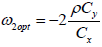we getSubstituting (3.4) (3.6) for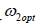we get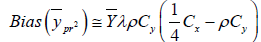(2.14)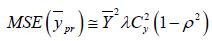(2.15)

Theoretical Comparison of Proposed Estimators

Following are the conditions under which the suggested estimator performs well than the existingestimators considered here.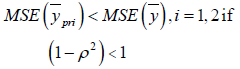Which is always true if and only if ρ ≠ 0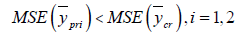IfThis is always true,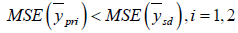If,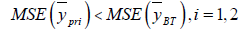If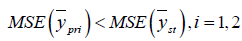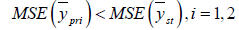IfIf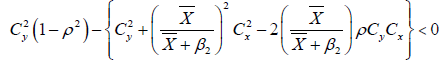Obviously the above conditions will always true when we apply it to real data sets.

Applications in SRS

Here in this section we will apply our proposed estimator to different real data sets taken from variousfield of life. The table 2 below shows that our proposed estimator is best as compared to the existingestimators, discussed in the literature. The following data sets have been considered for the comparisonpurpose.

Parameters data set 1 Source: Murthy (1967), Data set 2 Source: Murthy (1967), Data set 3 Source: US Agriculture Statistics(2010) Data set 4 Source: Koyuncu and Kadilar (2009) Data set 5 Source : Pakistan MFA (2004)
N 108 80 69 923 97
n 16 20 17 180 25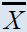461.3981 11.2664 4505.16 11440.498 3050.28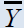172.704 51.8264 4514.9 436.43 3135.62
ρ  0.828315 0.3542 1.3756 1.718299 2.302173
ρ 0.6903 0.7507 1.18324 1.8645 2.327893
ρ 0.7896 0.9513 0.902327 0.9543 0.9871
β1  1.3612 1.05 5.141563 3.9365 28.345
β2 1.6307 -0.06339 29.77932 18.7208 50.32

Table 1: Differeent data sets with their parameteres values.

Estimators Population 1 Population 2 Population 3 Population 4 Population 5100 100 100 100 100263.83 66.28 439.899 939.7 3818.46263.92 82.5 440.15 940.11 3823.8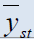168.81 200.13 448.38 817.15 895.53263.93 87.067 440.05 939.91 3820.9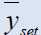264.03 65.05 448.34 943.8 3895.86266.15 877.54 538.19 1119.7 3994.77

Table 2: Percentage relative efficiency of the proposed estimators against some existing estimators

#### Conclusion

It is clear from above table that efficiency of our proposed estimators is optimum than all estimatorsconsidered in the literature, for all data sets. The conditions mentioned above also supported by the real data. Both estimators are equally efficient and give best results tan all others considered above. So we can modify some basic ratio estimators by assigning some suitable constants to them and hence their efficiency can be increases considerably.